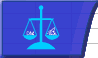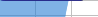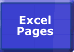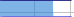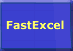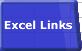FastExcel V3 ProfilerDrillDown Wizard Profile Workbook Profile Worksheet Profile Formulas FastExcel Whats New Purchase Licenses Volume Prices Upgrade from FastExcel V2 Installing FastExcel FastExcel Support FastExcel FAQ FastExcel LicenseFastExcel V3 Profiler

FastExcel Profiler is one of the FastExcel V3 family of products.

• Use FastExcel V3 Profiler to profile the calculation of your Excel workbooks to show you where the slow calculations are. human hair uk
• Use SpeedTools state-of-the-art tools to help you speed up slow Excel calculation.

Most slow-calculating workbooks have a small number of calculation bottlenecks. So the first step in speeding up calculation is to find these bottlenecks. You can use FastExcel V3 Profiler to drill-down to yourcalculation problems and prioritise them: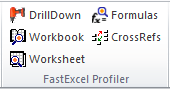Profile Workbook - detailed profiling of your workbook

Profile workbook provides and documents a detailed and prioritised analysis of the workbook:

• Analysis of the physical environment, workbook and calculation settings
• For the workbook and each worksheet
• Full calculation and recalculation times
• Volatility analysis
• Memory Useage analysis
• Used Range waste analysis
• Sparsity analysis
• Statistic counts of
• Used Range Cells
• Constants
• Conditional Formats
• Formulas
• Shapes
• Potential problem areas are highlighted in orange

Profile Worksheet - detailed profiling of a worksheet

Profile worksheet provides a detailed and prioritised analysis of a worksheet:

• For each Area, column group and row group
• Counts of Formulas, Array Formulas and Conditional Format Rules
• Calculation times compared to worksheet calculation times
• Potential problem areas are highlighted in orange

Profile Formulas - detailed profiling of the formulas on a worksheet

The ability to directly profile formulas is one of the major advances in FastExcel V3.
Profile formulas provides a detailed and prioritised analysis of each unique formula and function used on the worksheet:

• Count of the ocurrences of this formula
• Microseconds per formula
• Total calculation time for this formula compared to the worksheet
• Analysis of the formula
• Volatile or non-volatile
• Function types used (Native, UDF, XLL)

Drill Down Wizard - the easy way to find your calculation bottlenecks

• Step-by-step profiling drill down using defaults
• Finds the slowest worksheet in the workbook
• Then finds the slowest areas on the slowest sheet
• Then finds the slowest formulas on the slowest sheet

Map Cross-References - see the links between worksheets

• Shows forward and backward references between the worksheets in a workbook
• Option to minimise the forward references

Try it for yourself!

Note: the trial version of FastExcel V3 profiler does not enable Profile Workbook, Profile Worksheet and Profile Formulas, and the Drill down wizard will only profile a single worksheet.

You can convert the trial version of FastExcel V3 to a fully licensed version at any time by purchasing one of the FastExcel V3 licensing options.

Want to know more?

Download the FastExcel V3 Help file or the FastExcel V3 User Guide (PDF)© 2001-2014 Decision Models Legal Disclaimer Privacy Statement